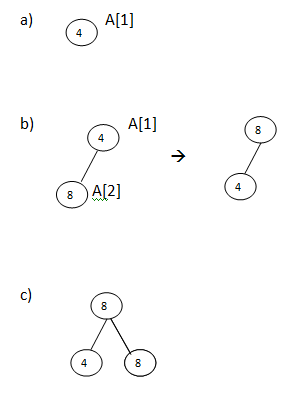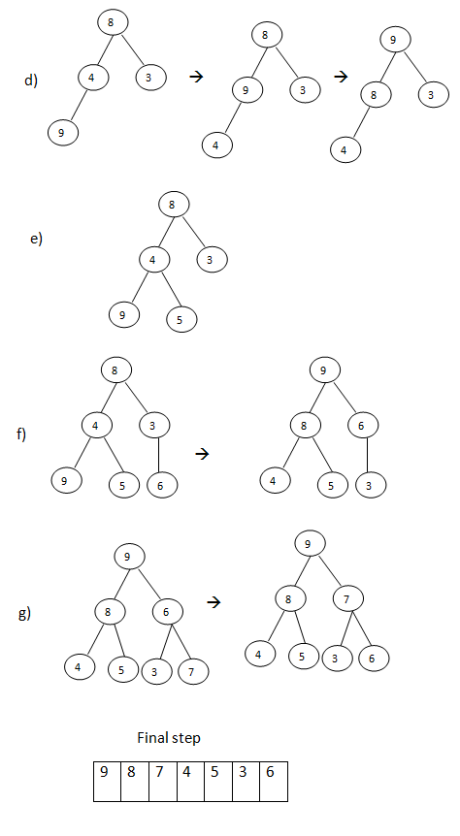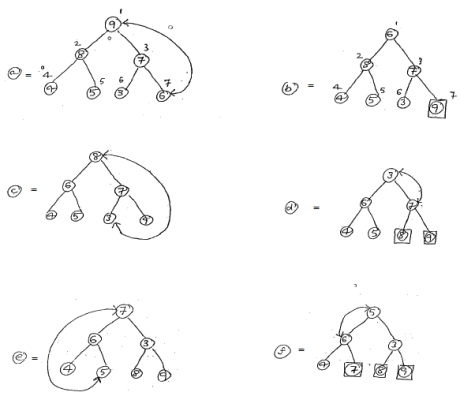# Heap Sort in Data Structures

21 September, 2018

Ratings

## Heap Sort

Building Heap

1. start with just one, element one element will always, satisfy heap property.
2. Insert next elements and make this a heap
3. Repeat step b until all elements are include in the heap

Sorting

1. Exchange the root name the last element in the heap
2. Make this a heap agar, but this time do not include the last node.
3. Repeat step a and b until there is no element left

#### Example

set the following elements in ascending order using heap sort 4,8,9,5.6   A

 4 8 3 9 5 6 1 2 3 4 5 6

### Build Heap### SortingProgram : Implementation of Heap Sort # include<stdio.h> void Heapsort(int a[],int n); void Build Heap(int a[],int n); void Reheap(int a[],int k); void main() { in a,n,i; printf(“Enter N value \n”); scanf(“%d”,&n); printf(“Enter those numbers: \n”); for(int i=1; i<=n; i++) { scanf(“%d”,&a[i]); } Heap sort(a,n); printf(“ The following are the sorted elements. \n”); for(i=1;i<=n;i++) { printf(“%d”,a[i]); } } void Heap sort(int a[],int n) { int fs,temp; Build Heap(a,n); for(pos=n;pos>=2;pos--) { temp=a; a=a[pos]; a[pos]=temp; Reheap(a,pos-1); } } void Build Heap(int a[],int n) { for(j=2;j<=n;j++) { key=a[i]; i=j/2; while((i>0) && (a[i]<key)) { a[j]=a[i]; j=i; i=j/2; } a[j]=key; } a[j]=key; } } void Reheap(int a[], int k) { int parent, child, key, heap; parent=1; child=2; Key=a[parent]; heap=0; while((child<=k) && (!heap)) { if(child<k) { if(a[child+1]>a[child]) child=child+1; } if(a[child]>key) { a[parent]=a[child]; parent=child; child=parent*2; } else { heap=1; } } a[parent]=key; }

### Time complexity :

Since each phase require time proportional to nlog2n, The running time to sort the n-element array using heap sort is proportional to nlog2n, that is f(n)=0(nlog2n) the average running time of heap sort is o(nlog2n)